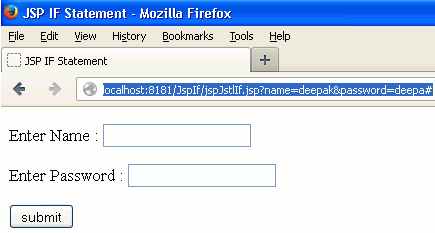Tutorials

# JSP IF Statement

If statement provides facility to apply the conditional statements in the programming language.

If statement provides facility to apply the conditional statements in the programming language.

## JSP IF Statement

In this section we will read about how the If statement is used in the JSP programming.

In JSP, If statement can be used as it is used in the core Java programming. Additionally, in JSP the conditional if statement can be implemented through JSTL. JSTL core tag library contains a tag <c:if> which is used to apply the if statement in JSP. Tag <c:if> is just work as the conditional IF statement in computer programming but, their syntaxes are different. Functionality of this conditional statement is to execute the sequence of statements of its body only when the expression given as the condition, evaluates to true.

Here we will use both IF statement in JSP.

Syntax of If statement

In computer programming language the IF statement is used in various ways. The IF statement can be used standalone, nested if statement, it may be used with the ELSE statement as well like IF-ELSE statement and/or as the IF-ELSE statement and/or IF-ELSE ladder statement.

In Java we can use the standalone IF statement, nested IF statement, IF-ELSE statement, nested IF-ELSE statement, IF-ELSE- ladder statement.

Simple IF statement syntax

```if(condition)
{
//statement
}
```

Nested IF statement syntax

```if(condition 1)
{
if(condition 2)
{
if(condition 3)
{
if(condition n)
{
//statement
}
}
}
}

```

IF-ELSE statement syntax

```if(condition)
{
//statement
}
else
{
//statement
}```

Nested IF-ELSE statement syntax

```if(condition 1)
{
if(condition 2)
{
//statement
}else  {
//statement
}
}else {
//statement
}
```

```if(condition 1)
{
//statement
}
else if(condition 2)
{
//statement
}
else if(condition n)
{
//statement
}
else
{
//statement
}
```

Syntax of JSTL Core <c:if> tag

As we have discussed earlier we can use the if statement using JSTL core tag <c:if> in addition to the JSP page. Here is the syntax of this tag to use on the JSP page.

```<c:if test="expression" var="variable" scope="scope">
//statement
</c:if>

```

In the syntax of the above <c:if> tag the attribute test is the required attribute and its value must be the 'boolean expression' other two attributes i.e. var, scope are optional value of these attributes must be of java.lang.String type.

Example

Here I am giving a simple example which will demonstrate you about how to use the IF statement in JSP page. Here we will see two JSP pages which will produce the same output but, both of the pages are created differently. From the two JSP pages first one is created using the JSTL core <c:if> tag that shows the name which has given into the textbox only if the name and password is matched and the second one JSP page is created using the standard IF statement that shows the name which has given into the textbox only if the name and password is matched.

Directory StructurejspJstlIf.jsp

```<%@ page language="java" contentType="text/html; charset=ISO-8859-1"
pageEncoding="ISO-8859-1"%>
<!DOCTYPE html PUBLIC "-//W3C//DTD HTML 4.01 Transitional//EN" "http://www.w3.org/TR/html4/loose.dtd">
<%@ taglib uri="http://java.sun.com/jsp/jstl/core" prefix="c" %>
<html>
<meta http-equiv="Content-Type" content="text/html; charset=ISO-8859-1">
<title>JSP IF Statement</title>
<body>
<form action="#">
<p>Enter Name :
<input type="text" name="name"/></p>
<p><input type="submit" value="submit"/></p>
</form>
<%
String name = request.getParameter("name");
%>
<!--JSP IF implementation using JSTL core if tag.-->

<c:set var="nm" value="<%=name %>"/>
<c:if test="\${(nm == 'deepak') && (psw == 'deepak')}">
<p>Welcome, <c:out value="\${nm }"/></p>
</c:if>
</body>
</html>
```

jspIf.jsp

```<%@ page language="java" contentType="text/html; charset=ISO-8859-1"
pageEncoding="ISO-8859-1"%>
<!DOCTYPE html PUBLIC "-//W3C//DTD HTML 4.01 Transitional//EN" "http://www.w3.org/TR/html4/loose.dtd">
<%@ taglib uri="http://java.sun.com/jsp/jstl/core" prefix="c" %>
<html>
<meta http-equiv="Content-Type" content="text/html; charset=ISO-8859-1">
<title>JSP IF Statement</title>
<body>
<form action="#">
<p>Enter Name :
<input type="text" name="name"/></p>
<p><input type="submit" value="submit"/></p>
</form>
<%
String name = request.getParameter("name");
%>
<!--JSP IF implementation.-->
<%
if(!(name == null || (name.equals(""))))
{
%>
<p>Welcome, <%=name %></p>
<%
}
%>
</body>
</html>

```

Output

When you will compile and deploy the above two JSP pages one by one then the output will be as follows :When you will provide the correct name and password i.e. name = 'deepak' and password = 'deepak' then the output will be as follows :But, when you will provide other than the value of either name or password to 'deepak' then nothing will be displayed on your page. In this output I have provided the wrong password (see in the address bar of browser) so there will be nothing displayed on the web page.